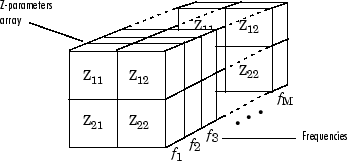# Z-Parameters Passive Network

Model passive network using Z-parameters

• Library:
• RF Blockset / Equivalent Baseband / Black Box Elements

•## Description

The Z-Parameters Passive Network block models a two-port passive network described in terms of its Z-parameters and their associated frequencies.

In the Z-Parameters field of the block dialog box, provide the Z-parameters for each of the M frequencies as a 2-by-2-by-M array. In the Frequency field, specify the frequencies for the Z-parameters as an M-element vector. The elements of the vector must be in the same order as the Z-parameters. For example, the following figure shows the correspondence between the Z-parameters array and the vector of frequencies.The Z-Parameters Passive Network block uses the RF Toolbox™ `z2s` function to convert the Z-parameters to S-parameters, and then interpolates the resulting S-parameters to determine their values at the modeling frequencies. The modeling frequencies are determined by the Output Port block. See Map Network Parameters to Modeling Frequencies for more details.

## Parameters

expand all

### Main

Z-parameters for a two-port passive network, specified as a 2-by-2-by-M array where M is the number of Z-parameters.

Frequency of Z-parameters, specified as an M-element vector with each element unit in hertz. All frequencies must be positive.

Method to interpolate the network parameters, specified as one of the following:

MethodDescription
`Linear`Linear interpolation
`Spline`Cubic spline interpolation
`Cubic`Piecewise cubic Hermite interpolation

### Visualization

Frequency data source, specified as ```Same as the Z-Parameters``` or `User-specified`.

Frequency data range, specified as a vector with each element unit in hertz.

Type of data plot to visualize using the given data, specified as one of the following:

• `X-Y plane` — Generate a Cartesian plot of the data versus frequency. To create linear, semilog, or log-log plots, set the Y-axis scale and X-axis scale accordingly.

• `Composite data` — Plot the composite data.

• `Polar plane` — Generate a polar plot of the data. The block plots only the range of data corresponding to the specified frequencies.

• `Z smith chart`, ```Y smith chart```, and `ZY smith chart` — Generate a Smith® chart. The block plots only the range of data corresponding to the specified frequencies.

Type of S-Parameters to plot, specified as one of the following. When noise is spectral, `NF` plotting is possible.

 `S11` `S12` `S21` `S22` `GroupDelay` `GammaIn` `GammaOut` `VSWRIn` `VSWROut` `OIP3` `IIP3` `NF` `NFactor` `NTemp` `TF1` `TF2` `TF3` `Gt` `Ga` `Gp` `Gmag` `Gmsg` `GammaMS` `GammaML` `K` `Delta` `Mu` `MuPrime`

Type of S-Parameters to plot, specified as one of the following. When noise is spectral, `NF` plotting is possible.

 `S11` `S12` `S21` `S22` `GroupDelay` `GammaIn` `GammaOut` `VSWRIn` `VSWROut` `OIP3` `IIP3` `NF` `NFactor` `NTemp` `TF1` `TF2` `TF3` `Gt` `Ga` `Gp` `Gmag` `Gmsg` `GammaMS` `GammaML` `K` `Delta` `Mu` `MuPrime`

Plot format, specified as one of the following.

 `dB` `Magnitude (decibels)` `Abs` `Mag` `Magnitude (linear)` `Angle` `Angle(degrees)` `Angle(radians)` `Real` `Imag` `Imaginary`

Plot format, specified as one of the following.

 `dB` `Magnitude (decibels)` `Abs` `Mag` `Magnitude (linear)` `Angle` `Angle(degrees)` `Angle(radians)` `Real` `Imag` `Imaginary`

Frequency plot, specified as `Freq`.

Frequency plot format, specified as one of the following.

 `Auto` `Hz` `kHz` `MHz` `GHz` `THz`

Y-axis scale, specified as `Linear` or `Log`.

X-axis scale, specified as `Linear` or `Log`.

Plot specified data using the plot button.